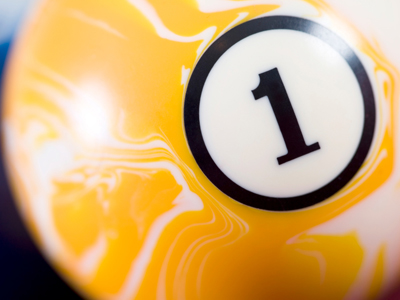Rapid Revision For School StudentsThe number 1. Can you add and take away 1 from other numbers?

# Year 1 Numbers - Order of Numbers

This quiz addresses the requirements of the National Curriculum KS1 Maths and Numeracy for children aged 5 and 6 in year 1. Specifically this quiz is aimed at the section dealing with recognising one more or one less than a given number.

Finding one more or one less than is all about understanding the order of numbers and knowing where they are in relation to each other. It's about being able to confidently count forwards or backwards and being able to add or subtract one from a number by counting up or down and knowing what is one more or one less than a given number.

Question 1
What is one less than 21?
20
22
12
2
One less means taking one away from the units digit
Question 2
What is one more than 79?
97
80
18
8
Counting up out loud can sometimes help
Question 3
What is one more than 16?
61
15
17
62
Imagine the number line in your mind and think about which numbers are next to each other
Question 4
What is one less than 100?
9
99
19
101
100 is the last number on a hundred square. 99 comes just before it, so is one less
Question 5
What is one less than 56?
55
57
45
65
Try to picture the numbers sitting on a number line
Question 6
What is one more than 100?
111
110
99
101
One more means adding one more to the units digit
Question 7
What is one more than 77?
88
78
87
77
One more means adding on one by counting up
Question 8
What is one less than 50?
94
59
40
49
Counting up to get to the given number will help to work out one less
Question 9
What is one more than 27?
27
26
28
78
Looking at the last digit is often helpful
Question 10
What number is one more than 0?
0
2
10
1
If you start at zero and count up, the first number you come to is one more than zero
Author:  Angela Smith